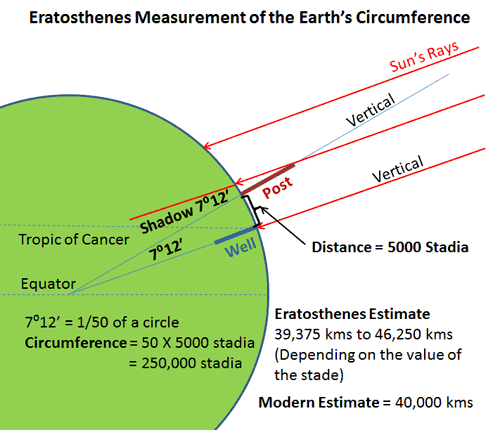Today we have thousands of satellites orbiting the Earth and through which we knew the shape of our planet and we calculated its circumference, the circumference of our blue planet extends for more than 40,000 kilometers from the east to the west, this number thousands of scientists and engineers spent decades from the last century in developing the techniques necessary for its calculation, It was discovered by a person in Egypt 2,000 years ago.

The story begins in Alexandria more than 2000 years ago with a Libyan man of Greek origin, whose name is Eratosthenes He hinted at a strange phenomenon. He received news that on the afternoon of a specific day of the year, the shadows disappear in the city of Aswan in Egypt or more precisely that the shadows retract until they disappear under the buildings, meaning that the sun is completely vertical over these buildings and this phenomenon occurs on June 24 of each year. It is known as the summer solstice: it is the day that marks the middle of the summer, the sun reaches its highest point, and the day is long.

Eratosthenes He seemed to wonder if the shadow would disappear in Aswan on June 21. Will it disappear in Alexandria? If the land is flat, the shadow will disappear in both cities.
So he waits on the 21st of June so he planted a stick in the city of Alexandria and started watching and noticed that the stick has a shadow tilted to the angle of 7.2, so this screaming could not happen. If the Earth is flat then the only explanation is that the Earth’s surface is curved, that his conclusion was logical, If the Earth’s surface were flat, the shadows would be equal in both cities, but since the Earth’s surface is curved, the lengths of the shadows will be different because the sun’s rays strike things from different angles.

The scientist has concluded The lengths of shadows differ between the two cities by 7.2 degrees. If we draw a line from Aswan to the center of the earth and from the city of Alexandria to the center of the earth, the angle will be 7.2°.

He said if I knew the distance between Aswan and Alexandria, I would be able to calculate the circumference of our planet, so he paid a sum of money to a person and asked him to calculate the distance between the two cities, so the man did that and estimated the distance by 800 kilometres, then the scientist got all the necessary data to calculate the circumference of the planet.The mathematical operation the world performed to calculate the circumference of our planet.

He learned after calculating the data that the circumference of the earth is equal to 40,000 km, but the actual circumference of the earth is 40.075 km, but this scientist and mathematician did a unique job, so he calculated the circumference of the earth using only a stick and his mind!

Thank you Eratosthenes.